### Home > PC > Chapter 8 > Lesson 8.1.2 > Problem8-27

8-27.
1. As stated above, in a recursive sequence each term is defined in terms of one or two (or, very rarely, more) of the terms immediately preceding. Practice this idea by using the first term and the recursive definition to write the next four terms of each of these sequences. The first one is partially done for you. Homework Help ✎

1. xn+1 = 4xn − 10; x1 = 5 [ x2 = 4x1 − 10 = 4(5) − 10 = 10; x3 = 4x2 − 10 = 4(10) − 10 = 30; x4 = ?; x5 = ?]

2. yn+1 = (yn)2 − 4; y1 = 0

3.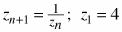4. wn+2 = wn+1 · wn ; w1 = 3, w2 = 5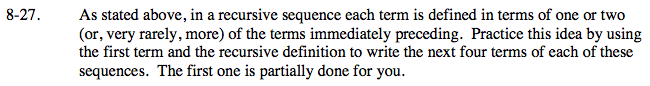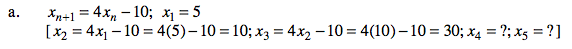x4 = 4(30) − 10

x5 = 4(4(30) − 10) − 10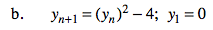y2 = 02 − 4
y3 = (02 − 4)2 − 4
y4 = ?
y5 = ?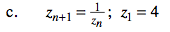$z_2=\frac{1}{4}$

$z_3=\frac{1}{\frac{1}{4}}$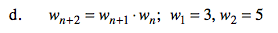w3 = w2 · w1 = 3 · 5